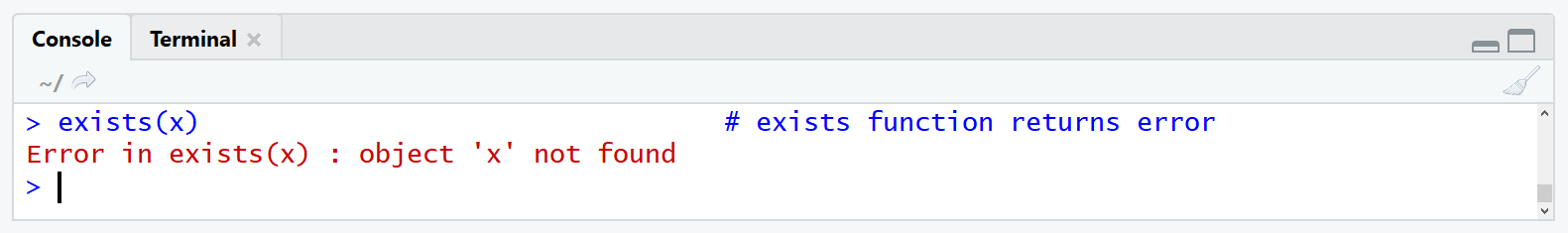# Check if Object is Defined (exists in R) | 4 Examples: Vector, Variable, Function, Error

In this R tutorial, I’ll show you how to check whether a data object exists in your R programming environment. The tutorial is mainly relying on the usage of the exists R function. Let’s start with the basic R syntax and the definition of the exists function:

Basic R Syntax:

`exists(x)`

Definition:

The exists function checks whether an R object is defined in the R environment.

The exists function is very flexible and can be applied to different R objects such as vectors, variables of a data.frame, or functions. In the following article, I’m going to show you four examples for the usage of exists.

Let’s dive into it!

## Example 1: Apply exists() Function to Vector

Probably the easiest and most intuitive way to apply the exists function is when we use it for vectors. Let’s create a simple vector for our first example:

`x <- c(2, 9, 5, 3) # Create example vector`

And not let’s use exists() to check if this vector is properly defined in our R environment:

```exists("x") # Apply exists function to vector # TRUE```

The exists function returns TRUE to the RStudio console. In other words: Yes, the vector x exists.

For comparison, let’s also apply the exists function to a vector that we did not define before:

```exists("y") # Apply exists function to non-existent vector # FALSE```

The exists command returns FALSE, i.e. the vector y does not exist.

That’s it! With this simple code we can check the existence of any data element we want.

If you need more information on this example of the exists function, you may check out the following video of the Statistics Globe YouTube channel. In the video, I’m explaining Example 1 in some more detail:

However, when we want to check for the existence of variables in a data.frame, it get’s slightly more difficult. But that’s what I’m going to show you in the next example.

## Example 2: Check if Variable in Data Frame is Defined

Let’s first create a data frame for this example:

```data <- data.frame(x1 = c(3, 9, 9, 2, 2), # Create example data.frame x2 = c(7, 8, 1, 4, 1))```

If we want to check for the existence of certain column names in this data frame, we need to attach the data first…

`attach(data) # Attach data.frame`

…and then we can check for the existence of variable names in our data:

```exists("x1") # Apply exists to variable of data # TRUE```

x1 exists…

```exists("x3") # Apply exists to non-existent variable # FALSE```

…but x3 doesn’t.

Hint: Don’t forget to detach the data afterwards. If you keep the data attached it might lead to confusion in the following programming steps.

`detach(data) # Detach data.frame`

## Example 3: Check if Function Exists

The exists R command can also be used to check for the existence of functions. For instance, we can check whether the function rbind.fill of the plyr package is defined:

```exists("rbind.fill") # Apply exists to check for function # FALSE```

As you can see, the function is not defined.

Why? Because we didn’t install and load the plyr package:

```install.packages("plyr") # Install plyr package library("plyr") # Load plyr package to R```

Now let’s apply exactly the same code as before:

```exists("rbind.fill") # Apply exists to check for function # TRUE```

The exists function returns TRUE – rbind.fill is available now.

In the fourth example, I want to show you an error message that might appear when exists is used:

`exists(x) # exists function returns error`Did you find the mistake? We didn’t put the data object we are looking for in quotes (i.e. “x”). Let’s try this again with quotes:

```exists("x") # Apply exists function correctly # TRUE```

Looks good!

## Video: The R Environment

In this tutorial, we spoke a lot about the environment of the R programming language. However, in if you are an unexperienced R user, the concept of the R environment may be difficult to understand.

But no worries! In the following video of the Bioinformatics DotCa YouTube channel, the speaker explains the functionality of the R environment in detail. Enjoy the video and see you next time!